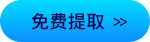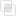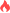python nan什么意思

Python2021-03-18 17:20:30837浏览 · 0收藏 · 0评论NaN是浮点数的一个值，代表“不是数”，通常是除0错误

python中可以用float("nan")或cmath.nan获得..

Python 中表示 Not A Number 使用小写的 nan

`a = float('nan')`

```from decimal import Decimal
a = Decimal('nan')```
```最常见的计算有 无穷大 减 无穷大 结果为 nan
float('inf') - float('inf')```

```import math
print(math.isnan(a))```

nan 和 nan 的比较关系是 nan != nan

（推荐操作系统：windows7系统、Python 3.9.1，DELL G3电脑。）初识Python-Python的历史与优缺点Python中文分词工具Python中文分词的原理你知道吗？什么是网络协议python中的去除重复项的操作python学习网

javascript字符串类型的转换419

PHP使用fread()操作字节447

PHP中define定义常量的方法434